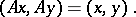# Unitary transformation

(diff) ← Older revision | Latest revision (diff) | Newer revision → (diff)

unitary mapping

A linear transformationof a unitary spacepreserving the inner product of vectors, i.e. such that for any vectorsandofone has the equalityA unitary transformation preserves, in particular, the length of a vector. Conversely, if a linear transformation of a unitary space preserves the lengths of all vectors, then it is unitary. The eigenvalues of a unitary transformation have modulus 1; the eigenspaces corresponding to different eigenvalues are orthogonal.

A linear transformationof a finite-dimensional unitary spaceis unitary if and only if it satisfies any of the following conditions:

1) in any orthonormal basis the transformationcorresponds to a unitary matrix;

2)maps any orthonormal basis to an orthonormal basis;

3) inthere exists an orthonormal basis of eigenvectors of, and, moreover,has in this basis a diagonal matrix with diagonal entries of modulus 1.

The unitary transformations of a given unitary space form a group under multiplication of transformations (called the unitary group).